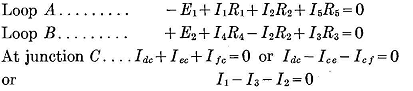Electrical Engineering is a free introductory textbook to the basics of electrical engineering. See the editorial for more information....# Network Solutions

Author: E.E. Kimberly

A circuit containing other than components in simple series or simple parallel is called a network. An example of a network is shown in Fig. 3-6. The currents and voltages in them are determined by the simultaneous applications of Kirchhoff's voltage and current laws.

In writing the path or loop equations for a network, such as that in Fig. 3-6, it is necessary that the directions taken around all paths be the same. After all the voltages have been found for all parts of the circuit, the voltage loop equations can be written for any closed traverse of the circuit. At any junction point in the circuit the currents in the branches connected to that point can be calculated by Kirchhoff's current law by so-called node equations.Fig. 3-6. Example of a Network

In Fig. 3-6 there are only three unknown currents designated as I1, I2, and I3, since I5 = I1 and I4 = I3. Therefore, there must be three equations. These may consist of two voltage equations and one current equation and are as follows:Also,It should be noted that the resistance voltage drop in a circuit is in the same direction as the current in it.

Example 3-6. - In Fig. 3-6, let E1 = 100, E2 = 90, R1 = 10, R2 = 8, R3=15, R4 = 20, and R5 = 30. Calculate the currents in and the voltage drops across all resistances.

Solution. - By Kirchhoff's voltage laws,

-100+10I1+8I2+30I5 = 0
+90+20I4-8I2+15I3 = 0

After simplification by substituting equivalent currents, these equations become:

48I1-8I3 = 100
-8I1+43I3 = -90

By the solution of these simultaneous equations,

I1 = +1.79 amp (Idc)
I3 = -1.76 amp (Ice or -Iec)

Then,

I2 = Icf = 1.79 - (-1.76) = 3.55 amp

The negative sign before 1.76, the value of I3, means that I3 is flowing in R3 in the direction opposite to that assumed when the loop B was traversed in a clockwise direction. Positive signs before the answers for I1 and I2 mean that the actual currents are in the same sense around the circuit as was assumed for loop A.

Last Update: 2010-11-30